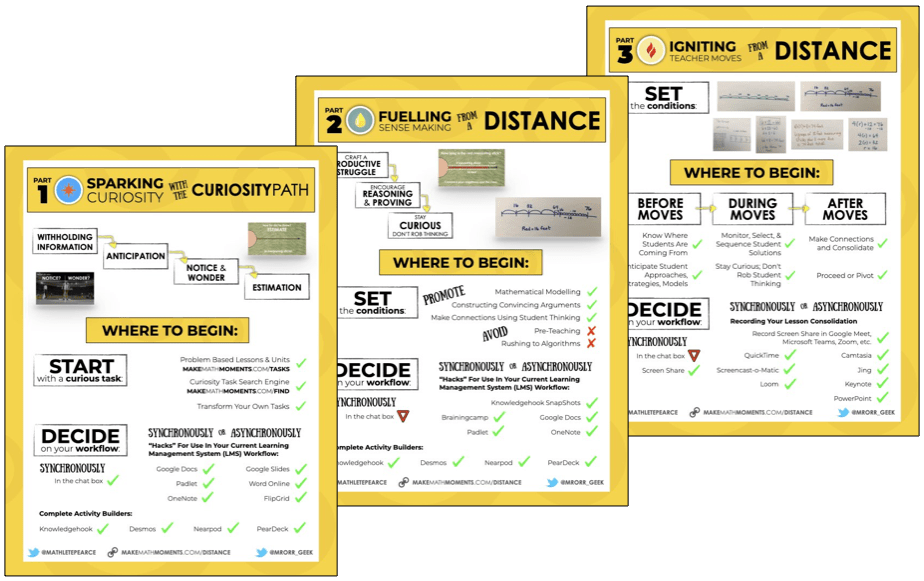# Solving Two-Step Equations Visually Part 2

In this series of Math Is Visual Prompts, we slowly introduce the idea of Solving Two-Step Equations Using Partitive and Quotative Division Scenarios.

In this series of Math Is Visual Prompts, students are given the opportunity to start solving two-step equations with both partitive and quotative division using typical word problems.

We also do our best to model this using the linear model of a number path (precursor to the number line) as well as abstractly using numbers and symbols.

As always, the visuals provided as a consolidation are useful AFTER students are given the opportunity to work with these ideas concretely and after consolidating in the classroom through student work.

Let’s get started…

## Math Is Visual Prompt #1:

The question is as follows:

After working a 4 hour shift, Sarah earned a total of \$45 including a \$5 tip. How much did she earn per hour?

So, let’s use a bar model to represent our thinking:Since Sarah’s \$45 in wages includes a \$5 tip, we’ll remove that tip in order to help us determine her hourly wage:Since she worked 4 hours and made \$40 in hourly wages, we can use partitive division to determine the rate or her hourly wage: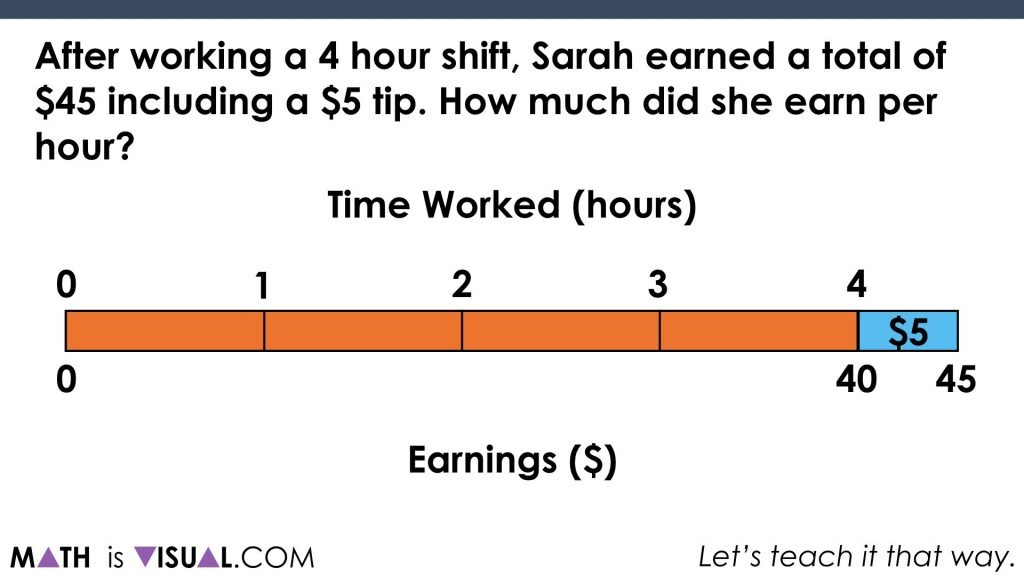We can see that when we partition \$40 into 4 groups (hours) she must have earned \$10 per hour: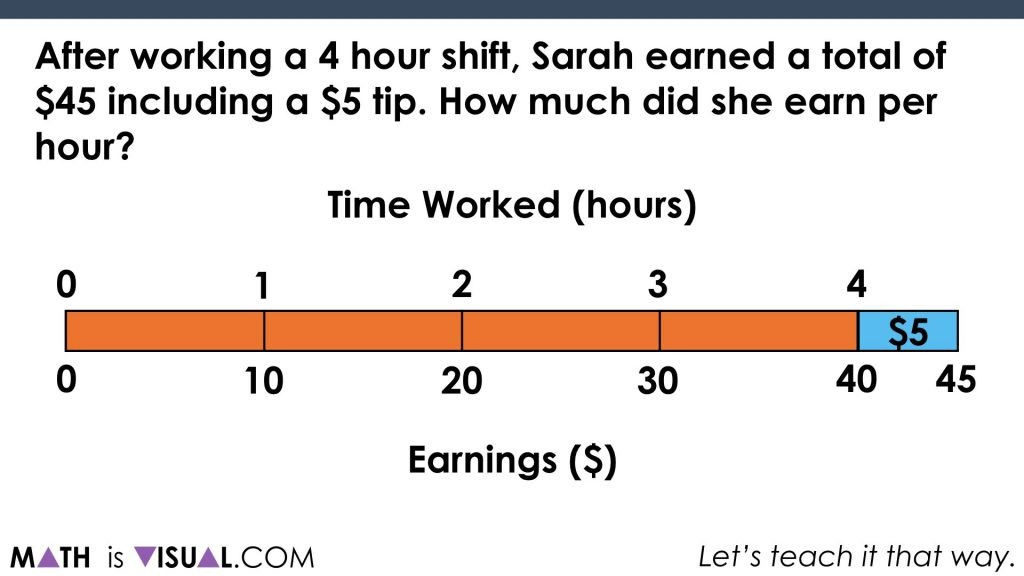We can see this process in the visual prompt video above algebraically as well:Visual Prompt #2:

In the second visual prompt, we are confronted with this word problem:

There are 19 people attending a dinner party seated at tables of 4 and one table of 3.
How many tables of 4 were there?

This time, we’ll use a concrete number line (linking cubes) to model out the 19 people:We will remove the constant (the single table of 3):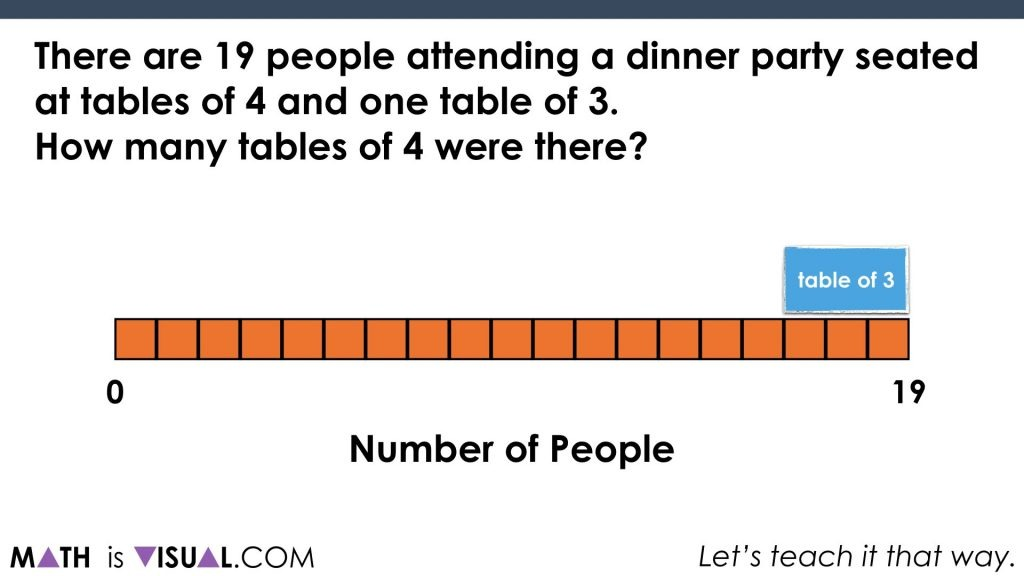Then, since we know our number of people at the tables of 4 (the rate per table or “quota” per group), we can use quotative division to determine how many tables of 4 there must be. This can be done through repeated subtraction (or addition, if you work your way up):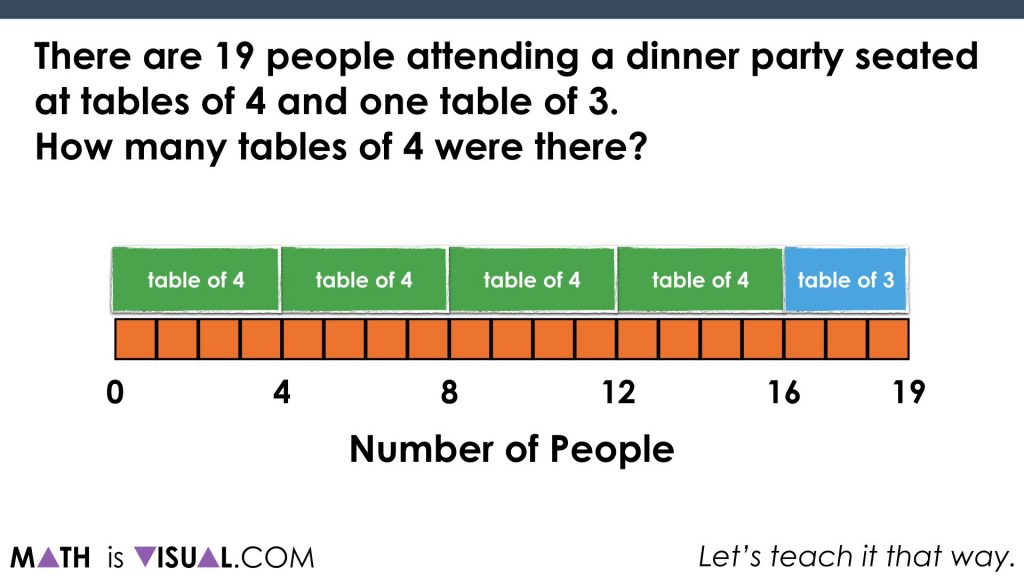Visual Prompt #3:

In the last word problem for this series of visual prompts, we are given the following:

Mr. Burke brings a group of 59 staff and students on a canoe trip. The participants on the trip will be using 3 person canoes and one 5 person canoe.

How many 3 person canoes will be used?

So, let’s use a number line for this particular representation and we’ll go ahead and subtract the constant (the single  5 person canoe):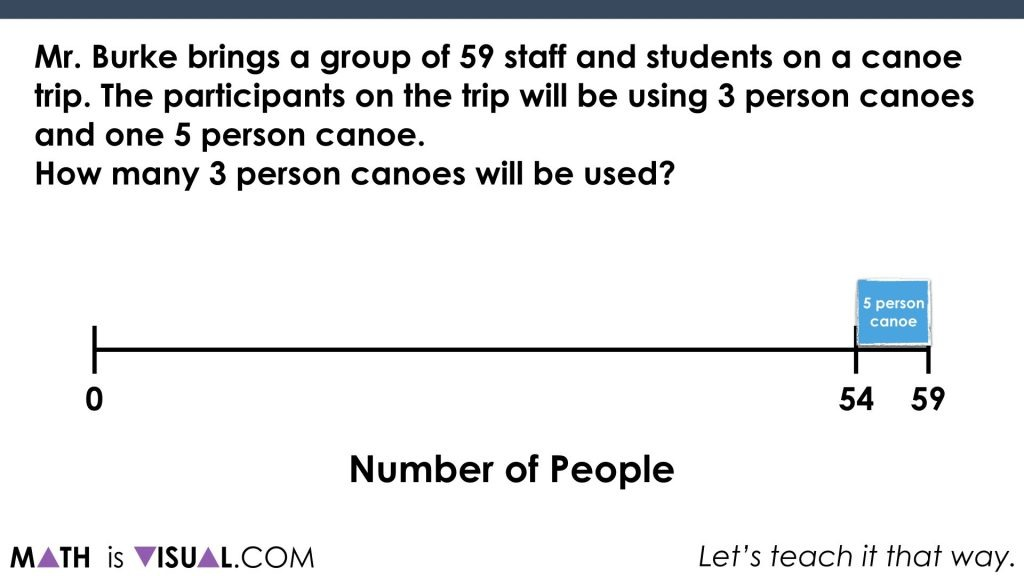In this example, you can determine the number fo 3 person canoes through quotative division again, however we are going to “scale up” rather than using repeated subtraction. This would be similar to a flexible division algorithm if you were working backwards: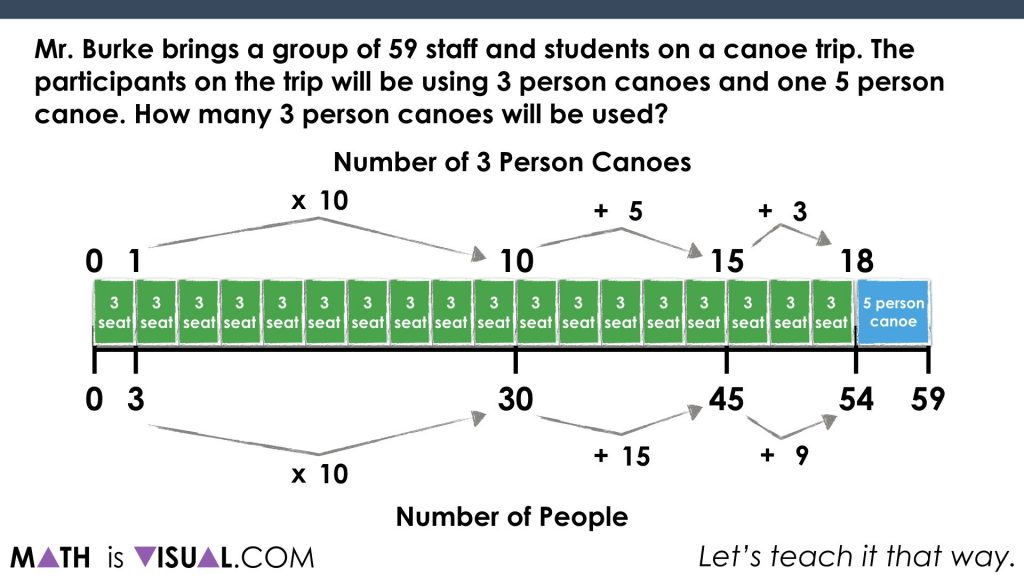Be sure to check out the visual prompts video at the top of the post to get the FULL EFFECT including “pause bars” to allow you to pause the video and let kids have think / discussion time.

How’d It Go?

Did you use this in your classroom or at home? How’d it go? Post in the comments!

Math IS Visual. Let’s teach it that way.#### kylepearce3